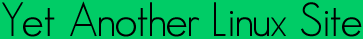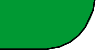Quick ?s
Cheat Sheets
Man Pages
The Lynx
Software
 ```COMPLEX(7) Linux Programmers Manual COMPLEX(7) NAME complex - basics of complex mathematics SYNOPSIS #include DESCRIPTION Complex numbers are numbers of the form z = a+b*i, where a and b are real numbers and i = sqrt(-1), so that i*i = -1. There are other ways to represent that number. The pair (a,b) of real numbers may be viewed as a point in the plane, given by X- and Y-coor dinates. This same point may also be described by giving the pair of real numbers (r,phi), where r is the distance to the origin O, and phi the angle between the X-axis and the line Oz. Now z = r*exp(i*phi) = r*(cos(phi)+i*sin(phi)). The basic operations are defined on z = a+b*i and w = c+d*i as: addition: z+w = (a+c) + (b+d)*i multiplication: z*w = (a*c - b*d) + (a*d + b*c)*i division: z/w = ((a*c + b*d)/(c*c + d*d)) + ((b*c - a*d)/(c*c + d*d))*i Nearly all math function have a complex counterpart but there are some complex-only functions. EXAMPLE Your C-compiler can work with complex numbers if it supports the C99 standard. Link with -lm. The imaginary unit is represented by I. /* check that exp(i * pi) == -1 */ #include /* for atan */ #include int main(void) { double pi = 4 * atan(1.0); complex z = cexp(I * pi); printf("%f + %f * i\n", creal(z), cimag(z)); } SEE ALSO cabs(3), carg(3), cexp(3), cimag(3), creal(3) COLOPHON This page is part of release 3.05 of the Linux man-pages project. A description of the project, and information about reporting bugs, can be found at http://www.kernel.org/doc/man-pages/. 2002-07-28 COMPLEX(7) ```Yals.net is © 1999-2009 Crescendo Communications Sharing tech info on the web for more than a decade! This page was generated Thu Apr 30 17:05:30 2009